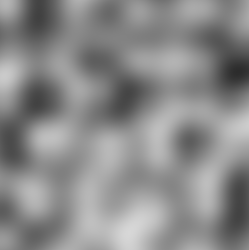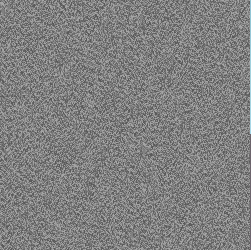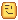# Basics of using Perlin Noise (2D Based)

Just typed it up in segments through the day at school

This is a tutorial for the 3D math function called perlin noise, used for “fog screens” and random terrain generation, and perlin worms.

What is the function

The function for perlin noise is very simple:

math.noise(X,Y,Seed)

X is your… X value, Y is your Y or Z value, and the seed is the seed used to calculate the numbers.

If the seed is greater than 0 and less than 1, the Y value will be between -1 and 1. It’s common range is -0.5 to 0.5, but does go beyond that range. If the seed is less than or equal to 0, or is greater than or equal to 1, it will always give 0.

So, what does this look like if you graph it?

As shown, the Seed will allow for different graphs to form each time. If the same seed is used, for each time the same X and Z are used, you will get the same number returned for your Y.

Fog Screens

The first thing one may want to ask is, what is a fog screen? This is one:They are blurry images that are generated with a given X and Z value, and use math.noise() to figure out the color. Example:

``````local ColorNum = (1 + math.noise(1,1,0.148))/2
Frame.BackgroundColor3 = Color3.new(ColorNum,ColorNum,ColorNum)``````

The issue is you need to use a massive amount of frames.

Problem Solving

Now, let us say you tried to do this for your first test, but got this:You may have this:

``````local X,Y = 1,4
local ColorNum = (1 + math.noise(X,Y,0.148))/2
Frame.BackgroundColor3 = Color3.new(ColorNum,ColorNum,ColorNum)``````

The issue is you are basically taking the numbers from each min and max on the graph.

To fix this, you can take a number in between the spikes and such by dividing the number.

``````local X,Y = 1,4
local ColorNum = (1 + math.noise(X/50,Y/50,0.148))/2
Frame.BackgroundColor3 = Color3.new(ColorNum,ColorNum,ColorNum)``````

The number you divide it by is relevant to what you want. It is good to play around, because the number could be 1, 200, 12, etc.

Terrain Generation:

Terrain generation is probably the most known topic for perlin noise, because it is so random. You could use sine waves, but they are not random. You pretty much just need to do the same as fog screens for terrain, in terms of have an X and Z, use math.noise(X/Scale,Y/Scale,Seed), then set your part’s position or terrain cell to X,Y * SomeScale (Unless you want the terrain to have a 2 unit difference as you go across, instead of mountains and valleys), Z.

Now, if you have a set minimum and max, and want to choose a position in that range, you can do this:

``````local Y = (1 + math.noise(X,Y,Seed)/2)
local Min,Max = 0,10
local NewY = Min + (Max - Min)*Y``````

The adding one to the number turns it’s range from -1 to 1 into 0 to 2, and dividing it by 2 will make its range 0 to 1, which can be used to lerp the two numbers.

Here are the differences when multiplying the Y.

Cave Generation/Perlin Worms:

Cave generation can be a seriously complex thing when you compare it to terrain. For using perlin noise, you would need to take 3 numbers then add it to the point, OR you can use math.random(-1,1), they will work the same if you go voxel based. Here is an example for finding points:

``````local X,Y,Z = 0,21,49
local XIn = math.noise(Y/Scale,Z/Scale,Seed)
local YIn = math.noise(X/Scale,Z/Scale,Seed)
local ZIn = math.noise(X/Scale,Y/Scale,Seed)
local NextPos = Pos + Vector3.new(X + XIn,Y + YIn,Z + ZIn)``````

Again, perlin noise is not needed if in the case you just do it on the server and do not want to follow a seed. If you want to do it on the client with a given seed, you can use this. Other alternatives like storing the info on the server do exist.

Example of outcome (by CloneTrooper1019):

Random Numbers:

Perlin Noise is used for random numbers, also known as psuedorandom. Basically, you can use the min and max, get a ratio from the noise function, and return the lerp behind them. Example code:

``````local X = 0

local function Random(Min,Max)
X = X + 1
local Ratio = (1 + math.noise(X,0,Seed)/2)
return Min + (Max-Min)*Ratio
end``````

Code to simulate math.noise (by CloneTrooper1019):

This more showing how it works, it is not in the client settings, so it does work offline.

``````local newMath = {}
local floor = math.floor
local perm = {}

for i = 1,512 do
perm[i] = math.random(1,256)
end

local function grad( hash, x, y )
local h = hash%8; -- Convert low 3 bits of hash code
local u = h<4 and x or y; -- into 8 simple gradient directions,
local v = h<4 and y or x; -- and compute the dot product with (x,y).
return ((h%2==1) and -u or u) + ((floor(h/2)%2==1) and -2.0*v or 2.0*v);
end

function PerlinNoise(x,y)
local ix0, iy0, ix1, iy1;
local fx0, fy0, fx1, fy1;
local s, t, nx0, nx1, n0, n1;
ix0 = floor(x); -- Integer part of x
iy0 = floor(y); -- Integer part of y
fx0 = x - ix0; -- Fractional part of x
fy0 = y - iy0; -- Fractional part of y
fx1 = fx0 - 1.0;
fy1 = fy0 - 1.0;
ix1 = (ix0 + 1) % 255; -- Wrap to 0..255
iy1 = (iy0 + 1) % 255;
ix0 = ix0 % 255;
iy0 = iy0 % 255;
t = (fy0*fy0*fy0*(fy0*(fy0*6-15)+10));
s = (fx0*fx0*fx0*(fx0*(fx0*6-15)+10));
nx0 = grad(perm[ix0 + perm[iy0+1]+1], fx0, fy0);
nx1 = grad(perm[ix0 + perm[iy1+1]+1], fx0, fy1);
n0 = nx0 + t*(nx1-nx0);
nx0 = grad(perm[ix1 + perm[iy0+1]+1], fx1, fy0);
nx1 = grad(perm[ix1 + perm[iy1+1]+1], fx1, fy1);
n1 = nx0 + t*(nx1-nx0);
return 0.5*(1 + (0.507 * (n0 + s*(n1-n0))))
end

function newMath.noise(seed,x,z)
local perlin = PerlinNoise(x,z+(seed*10000))
return -1 + (perlin *2)
end

local meta = {
__index = function (_,index)
local get = rawget(newMath,index)
if get then
return get
else
return math[index]
end
end;
}
setmetatable(newMath,meta)

return function ()
getfenv(2).math = newMath
end
``````

So that… is the basics of Perlin Noise.

And remember… you can do more than what this post shows…Want to share this? Here is a link to an edited image of this whole post that shows everything and links where necessary: Imgur: The magic of the Internet

76 Likes

Problem is, math.noise is 3D noise.

i.e. you use it like this:

``local density = math.noise(x, y, z)``

Density is the easiest way to visualise the value it returns, and it makes generation like Minecraft terrain possible. (i.e. the overhangs, etc.)

In fact, we do have two articles for math.noise on the wiki, too:

Regardless, using the z value as a seed is still a very clever way of using it and turning it into a 2D heightmap.

4 Likes

The weird thing was that I got numbers that were greater than 0.5.

I was wondering where the documentation was, because I could not find it using the search. :uhhh:

1 Like

[quote] The weird thing was that I got numbers that were greater than 0.5.

I was wondering where the documentation was, because I could not find it using the search. :uhhh: [/quote]

That’s strange because when you search “math.noise” you get this:
http://wiki.roblox.com/index.php?title=Math.noise&redirect=no

(I appended &redirect=no to not redirect you :P)

1 Like

The search gave me the Roblox specific functions, which did not even have the function.

1 Like

Is it possible if i can have the code for the perlin noise graph?

1 Like